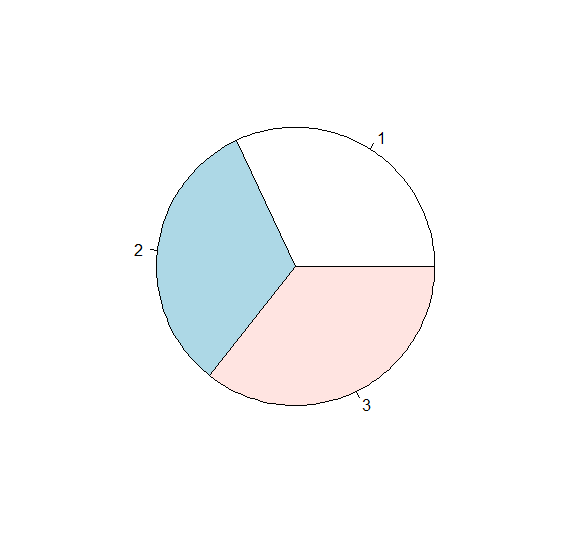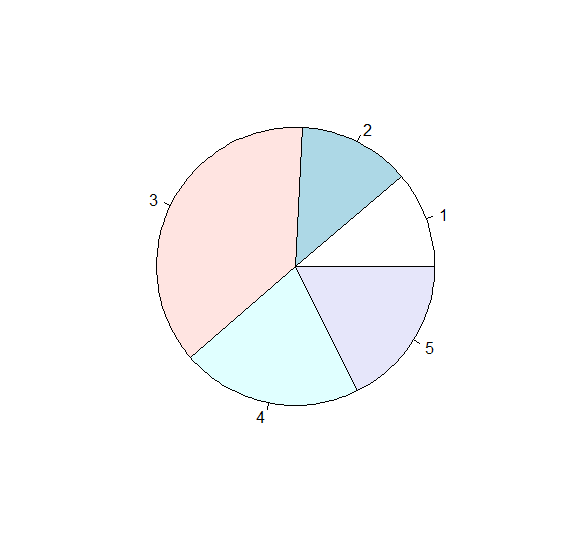# How to create pie chart in base R?

A pie chart is a circular representation of data which is created for either nominal data or ordinal data. The slices in the pie chart depend on the magnitude of the data values. If we want to create a pie chart in base R then pie function can be used.

For Example, if we have a vector called X then the pie chart for values in X can be created by using the below command −

pie(X)

## Example

Following snippet creates a sample data frame −

x<-sample(1:100,3)
pie(x)

## Output

If you execute the above given snippet, it generates the following Output −## Example

Following snippet creates a sample data frame −

x<-sample(1:100,3)
pie(x)
y<-c(24,28,80,45,38)
pie(y)

## Output

If you execute all the above given snippets as a single program, it generates the following Output −